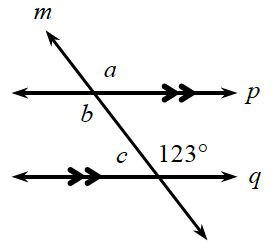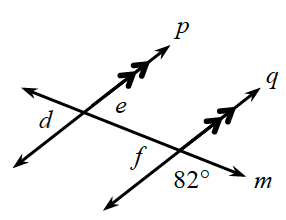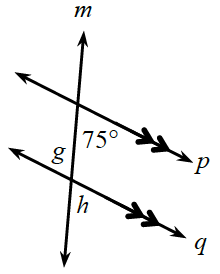### Home > CC4 > Chapter 1 > Lesson 1.2.1 > Problem1-42

1-42.

Recall what you learned about angle relationships in previous courses. (See the Math Notes box in this lesson if you need a reminder.) Determine the measures of the labeled angles. The double arrowheads indicate that the pair of lines is parallel. Homework Help ✎

All three of these can be solved using multiple techniques related to parallel lines.

1.$\angle a$: Corresponding angle with $123º$, so $m\angle a = 123º$ .
$\angle b$: $\angle a$ and $\angle b$ are vertical angles, so they have equal measures. Therefore, $m\angle b = 123º$.
$\angle c$: $\angle c$ and $123º$ form a pair of supplementary angles, so $m\angle c = 57º$.

1.$\angle f$ and $82º$ form a pair of supplementary angles. How are $\angle e$ and $\angle f$ related?

1.Since there are parallel lines, look for relationships between pairs of angles such as alternate interior, corresponding, or same-side interior.